# Texas Go Math Grade 1 Lesson 18.2 Answer Key Time to the Half Hour

Refer to our Texas Go Math Grade 1 Answer Key Pdf to score good marks in the exams. Test yourself by practicing the problems from Texas Go Math Grade 1 Lesson 18.2 Answer Key Time to the Half Hour.

## Texas Go Math Grade 1 Lesson 18.2 Answer Key Time to the Half Hour

Explore
Circle 4:00, 5:00, or between 4:00 and 5:00 to describe the time shown on the clock.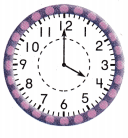4:00
between 4:00 and 5:00
5:00Explanation:
Time shown in the clock is 4’O clock.FOR THE TEACHER • Have children look at the hour hand on each clock to decide which choice best describes the time shown.
Hours hand on 12 and minutes hand on 4, shows the time as 4’O clock.

Explanation:
Hours hand on 12 and minutes hand on 4, shows the time as 4’O clock.

Math Talk
Mathematical Processes
Use before and after to describe the time, shown on the middle clock.

Model and Draw
As an hour passes, the hour hand moves from one number to the next number.
The minute hand points to 12.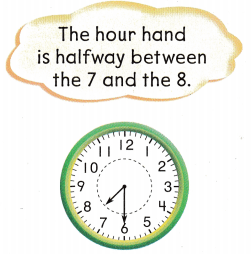When a half hour has passed, the hour hand points halfway between two numbers.
The minute hand points to 6.Share and Show
Look at where the hour hand points. Write the time.
Question 1.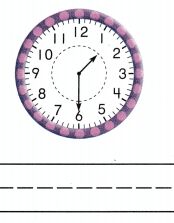Explanation:
When a half hour has passed 1, the hour hand points halfway between two numbers.
The minute hand points to 6.
Time shown is 1:30 or half past 1:00.

Question 2.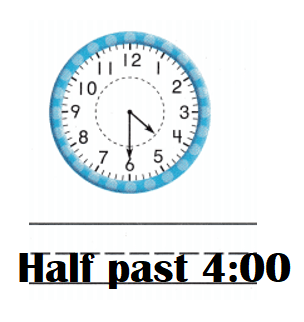Explanation:
When a half hour has passed 4, the hour hand points halfway between two numbers.
The minute hand points to 6.
Time shown is 4:30 or half past 4:00.

Question 3.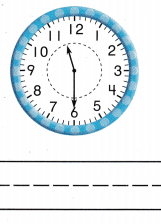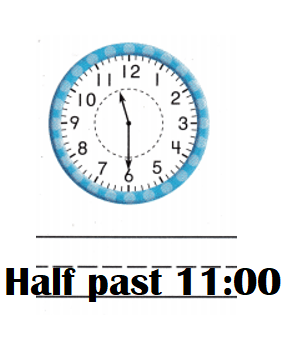Explanation:
When a half hour has passed 11, the hour hand points halfway between two numbers.
The minute hand points to 6.
Time shown is 11:30 or half past 11:00.

Question 4.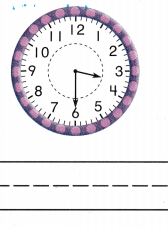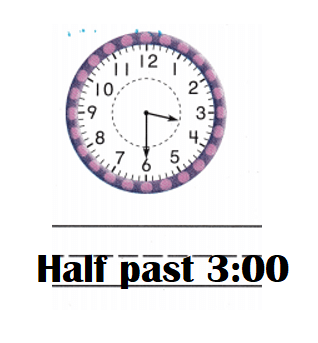Explanation:
When a half hour has passed 3, the hour hand points halfway between two numbers.
The minute hand points to 6.
Time shown is 3:30 or half past 3:00.

Problem Solving
Write the time.
Question 5.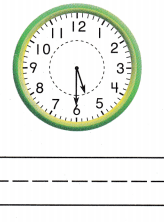Explanation:
Time shown in the clock is 5:30 or half past 5:00.

Question 6.Explanation:
Time shown in the clock is 10:30 or half past 10:00.

Question 7.
H.O.T. Tim plays soccer at half past 9:00. He eats lunch at half past 1:00. He sees a movie at half past 2:00.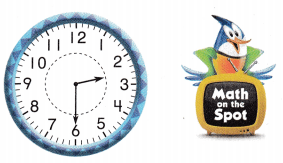Look at the clock.
Write what Tim does.
TimTim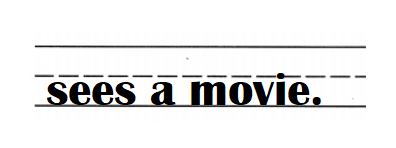Explanation:
Time at Tim plays soccer at half past 9:00.
He eats lunch at half past 1:00.
He sees a movie at half past 2:00.
=> Time shown in the clock is 2:30 or half past 2:00.

Question 8.
H.O.T. Multi-Step Tyra has a piano lesson at 5:00. The lesson ends at half past 5:00. How much time is Tyra at her lesson? Circle your answer.
half hour
hour
Time of Tyra at her lesson = 30 minutes.

Explanation:
Time at Tyra has a piano lesson = 5:00
Time at when the lesson ends = at half past 5:00.
=> 5:30.
Time of Tyra at her lesson = Time when the lesson ends – Time at Tyra has a piano lesson
= 5:30 – 5:00
= 30 minutes.

Question 9.
The game starts at the time shown on the clock. What time does the clock show?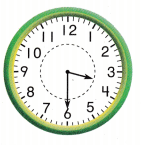(A) half past 4
(B) half past 5
(C) half past 3
Time shown in the clock is 3:30 or half past 3.
(C) half past 3.

Explanation:
Time shown in the clock is 3:30 or half past 3.Question 10.
Which clock shows half past 7?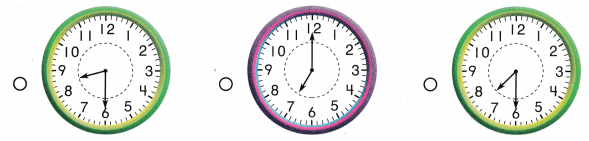Explanation:
First clock shows the time: 8:30 or half past 8.
Second clock shows the time: 7:00.
Third clock shows the time: 7:30 or half past 7.

Question 11.
Jeff starts reading at 8:30. Which shows the time Jeff starts reading?
(A) half past 7
(B) half past 8
(C) half past 9
Half past 8 shows the time Jeff starts reading.
(B) half past 8.

Explanation:
Time at Jeff starts reading = 8:30 or half past 8.

Question 12.
Texas Test Prep What time does the clock show?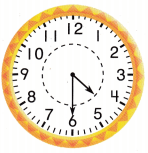(A) 5:00
(B) half past 4:00
(C) 4:00
Clock shows the time: 4:30 or half past 4.
(B) half past 4:00.

Explanation:
Clock shows the time: 4:30 or half past 4.

TAKE HOME ACTIVITY. Say a time, such as half past 10:00. Ask your child to describe where the hour hand points at this time.
My kids said Half past 8:30, the hour hand points at 6.

Explanation:
My kids said Half past 8:30, the hour hand points at 6.

### Texas Go Math Grade 1 Lesson 18.2 Homework and Practice Answer Key

Look at where the hour hand points. Write the time.
Question 1.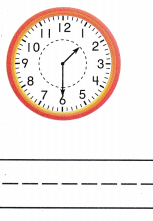Explanation:
Clock shows the time: 1:30 or half past 1.

Question 2.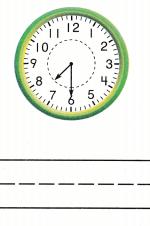Explanation:
Clock shows the time: 7:30 or half past 7.

Problem Solving
Question 3.
Kenny wakes up at 8:00. He visits a friend at half past 10:00. He goes to the playground at 1:00.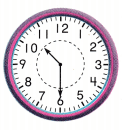Look at the clock. Write what Kenny does.
Kenny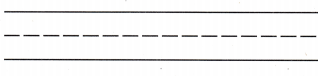Clock shows 10:30 or half past 10.Kenny visits his friend.
Kenny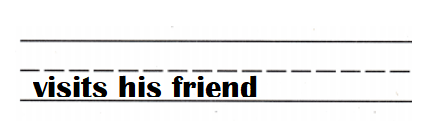Explanation:
Time at Kenny wakes up = 8:00.
Time at he visits his friend = 10:00.
Time at he goes to the playground = 1:00.

Lesson Check
Question 4.
The concert starts at the time shown on the clock. What time does the clock show?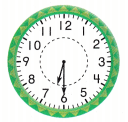(A) half past 7:00
(B) half past 8:00
(C) half past 6:00
The clock shows 6:30 or half past 6.
(C) half past 6:00.

Explanation:
The clock shows 6:30 or half past 6.Question 5.
Which clock shows half past 3?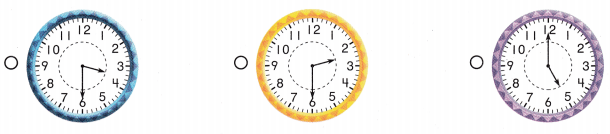Explanation:
First clock shows: 3:30 or  half past 3.
Second clock shows: 2:30 or half past 2.
Third clock shows: 5:00.

Question 6.
What is the time?(A) half past 10:00
(B) half past 9:00
(C) 9:00
The clock shows the time: 9:30 or half past 9.
(B) half past 9:00.

Explanation:
The clock shows the time: 9:30 or half past 9.Question 7.
Multi-Step Alex starts eating at 5:00. One half hour passes. What time does he stop eating?
(A) half past 4:00
(B) half past 5:00
(C) half past 6:00
Time he stops eating = 5:30 or half past 5.
(B) half past 5:00.

Explanation:
Time at Alex starts eating = 5:00.
One half hour passes = half of an hour = 1 ÷ 2 hour
=> 0.30 hour or 30 minutes.
Time he stops eating = Time at Alex starts eating + One half hour passes
= 5:00 + 0.30
= 5:30 or half past 5.

Scroll to Top